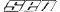Staredit Network > Forums > Null > Topic: Free kisses from me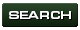Free kisses from me
Sep 11 2007, 12:35 am
By: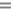MillenniumArmy

Sep 11 2007, 12:35 amMillenniumArmy Post #1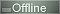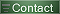Free kisses from me if you are the first one to find the solution of the given First order differential equation of the given initial value problem:

y'+2y = te^(-2t)
at y(1) = 0

gogogo

None.

Sep 11 2007, 12:35 am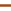blacklight28 Post #2Is this just like your homework or something?

None.

Sep 11 2007, 12:40 amMillenniumArmy Post #3Oh I already know how to do this. I just feel like giving someone an incentive to receive a nice kiss from me. And perhaps, even a personal shout out from me in my mini-game party map =-O

None.

Sep 11 2007, 12:41 amblacklight28 Post #4Well I have no clue what it is but I want a personal shout out from you anyway for trying!! PLZ!!

None.

Sep 11 2007, 1:02 am Akar Post #5whats the y' mean, is that just y?
damnit calculus! You asked 3 years too early >.>
Heres what I can do:
2y = 0
y = 0

y' + 2y = te ^ (-2t)
---------------------
2y
and thus no solution xD because y = 0

OR:

0' + 2(0) = te ^ (-2t)
0 = te ^ (-2t) < I'm assuming 0' is a function of multiplication
1/t = e ^ (-2t)
1/t ^ (-2t) = e < I'm guessing on this >.>
0 = t[ 1/t ^ (-2t)]
0 = 1 ^ (-2t)
I'm guessing I did that wrong becuase nothing in squares = 0.

Post has been edited 4 time(s), last time on Sep 11 2007, 1:22 am by Akar.

None.

Sep 11 2007, 1:49 amMillenniumArmy Post #6Quote from Akar
whats the y' mean, is that just y?
damnit calculus! You asked 3 years too early >.>
Heres what I can do:
2y = 0
y = 0

y' + 2y = te ^ (-2t)
---------------------
2y
and thus no solution xD because y = 0

OR:

0' + 2(0) = te ^ (-2t)
0 = te ^ (-2t) < I'm assuming 0' is a function of multiplication
1/t = e ^ (-2t)
1/t ^ (-2t) = e < I'm guessing on this >.>
0 = t[ 1/t ^ (-2t)]
0 = 1 ^ (-2t)
I'm guessing I did that wrong becuase nothing in squares = 0.
It means what it means; y prime.

And ur answer is nowhere even close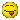clue: to solve this, you have to be able to know how to do integrals ;P

None.

Sep 11 2007, 1:51 am Akar Post #7never the less my first statement is correct, even if it isn't what your asking.

None.

Sep 11 2007, 2:22 am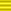DT_Battlekruser Post #8Yuck, I fail at isolating y and dy, t and dt.

Too tired to give it any really serious thought. (I assume y' = dy/dt)

EDIT ~ Looked a few things up online - I haven't had Differential Equations yet (or Calculus III). As far as I can tell, a simple two-semester knowledge of calculus isn't sufficient.

Post has been edited 1 time(s), last time on Sep 11 2007, 2:40 am by DT_Battlekruser.

None.

Sep 11 2007, 2:39 amMillenniumArmy Post #9Quote from Akar
never the less my first statement is correct, even if it isn't what your asking.
No even your very first statement is incorrect because you're not using the given info correctly. You're probably thinking that "y(1) = 0" means "y = 0." y(1) = 0 means that when the variable t equals 1, then the variable y equals 0, but you shouldn't even use this information after integrating the whole equation.

Quote
Yuck, I fail at isolating y and dy, t and dt.
It's not about isolating any of those you just mentioned; for problems like this you're suppose to only isolate the y' (or in other words dy/dt) but in this case it's already isolated, so the next thing you're suppose to do is to multiply both sides by an unknown function (which you have to figure out how to do) that will make the left side of the equation integrateable (meaning after integration y' will not exist)None.

Sep 11 2007, 2:41 amDT_Battlekruser Post #10Yeah, I looked it up, I haven't learned how to do that yet.

None.

Sep 11 2007, 2:42 amMillenniumArmy Post #11Well technically all you need is calculus BC to solve this. You only need to use differentials and mainly integrals to solve this. The thing is you just gotta know how to apply them lol

None.

Sep 11 2007, 2:45 amDT_Battlekruser Post #12Well, I'll wait for someone with more time to come solve itI'm sure I'll get it once I see the solution.

None.

Sep 11 2007, 2:45 amcheeze Post #13y + y^2 = integral of that

None.

Sep 11 2007, 2:54 am Akar Post #14damn foiled again.
a = b
a^2 = ab
a^2 -b^2 = ab - b^2
(a-b)(a+b) = b(a-b)
a+b = b
2b = b
2 = 1

None.

Sep 11 2007, 9:57 pm Dr. Shotgun Post #15Ask me next year.

None.

Sep 12 2007, 8:15 amMillenniumArmy Post #16Boo. No kisses from me then.

But anyways, here's the solution:

---------------------------------
y'+2y = te^(-2t)
at y(1) = 0
---------------------------------

y'+2y = te^(-2t)
becomes:

m(t)y'+m(t)2y = m(t)te^(-2t)

now m(t) is an unknown function, so we have to solve it so that the left side of the equation (the y'+2y) becomes integrateable and have the y' be eliminated so we do this:

m(t) = e^( S2dt) = e^(2t)
so now we have:

(e^(2t))y'+(e^(2t))2y = (e^(2t))te^(-2t)

now before you integrate both sides, you have to eliminate the y'. And to do this, you just do the product rule for differentiation backwards (this is not the same thing as integration). So you end up with:

(d/dt)ye^(2t) = t (the e^(2t) and e^(-2t) on the right side cancel each other out)

Now when you integrate both sides, you get:
ye^(2t) = (t^2)/2 + c

Isolate the y now and you get:
y = ((t^2)/2 + c)/(e^(2t))

------------------------------------

now at the beginning, we were given y(1) = 0. Basically this means that when t = 1, y is 0, so let's plug those into the new equation:
0 = ((1^2)/2 + c)/(e^2t))
solve for c algebraically, and you end up with:
c = -1/2
now replace c with -1/2 and you have your answer:

===================
y = (t^2 - 1)/(2(e^(2t)))
===================

None.

Sep 12 2007, 10:17 pmDT_Battlekruser Post #17It makes sense, but I was definitely never taught that.

Very crafty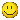How did you find m(t), or was it just looking and seeing it intuitively?

None.

Sep 12 2007, 11:14 pmSymmetry Post #18Dungeon MasterQuote from Akar
(a-b)(a+b) = b(a-b)

aa+ab-ab-bb = ba-bb

0=0? I think you expanded it wrong, bud.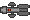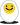Sep 13 2007, 9:04 pmMillenniumArmy Post #19Quote from DT_Battlekruser
It makes sense, but I was definitely never taught that.

Very craftyHow did you find m(t), or was it just looking and seeing it intuitively?
The equation in my very first post was in the form of:

dy/dt + ay = g(t)

so a was 2 in that case.

Well this is actually something new that was taught to us in class, but pretty much the trick to this is that to find m(t), you set m(t) = e^(integral of a)

This only works for simple equations like this, where it's already in the perfect form.

None.

Sep 14 2007, 1:34 pmParavin. Post #20Quote from MillenniumArmy
Boo. No kisses from me then.

But anyways, here's the solution:

---------------------------------
y'+2y = te^(-2t)
at y(1) = 0
---------------------------------

y'+2y = te^(-2t)
becomes:

m(t)y'+m(t)2y = m(t)te^(-2t)

now m(t) is an unknown function, so we have to solve it so that the left side of the equation (the y'+2y) becomes integrateable and have the y' be eliminated so we do this:

m(t) = e^( S2dt) = e^(2t)
so now we have:

(e^(2t))y'+(e^(2t))2y = (e^(2t))te^(-2t)

now before you integrate both sides, you have to eliminate the y'. And to do this, you just do the product rule for differentiation backwards (this is not the same thing as integration). So you end up with:

(d/dt)ye^(2t) = t (the e^(2t) and e^(-2t) on the right side cancel each other out)

Now when you integrate both sides, you get:
ye^(2t) = (t^2)/2 + c

Isolate the y now and you get:
y = ((t^2)/2 + c)/(e^(2t))

------------------------------------

now at the beginning, we were given y(1) = 0. Basically this means that when t = 1, y is 0, so let's plug those into the new equation:
0 = ((1^2)/2 + c)/(e^2t))
solve for c algebraically, and you end up with:
c = -1/2
now replace c with -1/2 and you have your answer:

===================
y = (t^2 - 1)/(2(e^(2t)))
===================

I think my brain just exploded.

None.

Options
Back to forum
Members in this topic: None.
[12:03 am]Pr0nogo -- Suicidal Insanity
Suicidal Insanity shouted: But dat files probably would be a massive pain to support, so unlikely I would ever do that
would be nice to at least have the option to edit player map settings for ids past the default limit, but I guess we have ways around that already
[11:01 pm]Corbo -- Suicidal Insanity
Suicidal Insanity shouted: Pr0nogo And custom color cycling !
and custom parallax support tbh
[10:43 pm]
Suicidal Insanity -- Suicidal Insanity
Suicidal Insanity shouted: CorboAnd statistics
[10:42 pm]
Suicidal Insanity -- jjf28
jjf28 shouted: Corbo qft
Lies !
[10:30 pm]
Suicidal Insanity -- Btw, did you try the build with the tileset fix?
[10:29 pm]
Suicidal Insanity -- But dat files probably would be a massive pain to support, so unlikely I would ever do that
[10:28 pm]
Suicidal Insanity -- Pr0nogo
Pr0nogo shouted: he's already added expanded tileset functionality which requires an external plugin
And custom color cycling !
[10:21 pm]jjf28 -- Corbo
Corbo shouted: no one quotes me :'(
qft
[09:30 pm]Corbo -- no one quotes me :'(
[07:33 pm]Pr0nogo -- meant to quote poiuy_qwert
poiuy_qwert shouted: There are some limit expander plugins floating around, at least one that allows expanded dat filesMembers Online:jjf28,Pr0nogo,Roy,Corbo